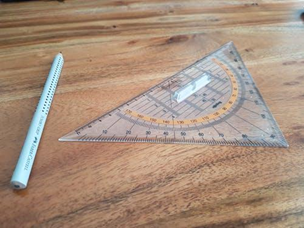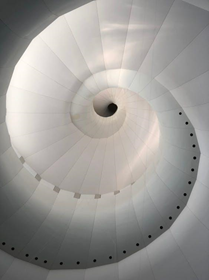Mathematics

# Geometry Study Guide: Learning Geometry the right way!

1.2k views

 1 Introduction 2 Geometry Study Guide: How to learn geometry the right way? 3 Summary 4 FAQs 5 External References

21 November 2020

## Introduction

What is geometry?

How to study geometry?

GEOMETRY- means “Earth Measurement” a rough translation from Greek. Look around you and you will see shapes and spaces everywhere. Your home, the arrangement of furniture in your room, the layout of the rooms, the placement of windows, the dining table, the plate from which you eat, even the food served to you is cut or made in a shape.

So, all around us, we see the dynamics between shapes and spaces. When you rush to the sports room in your school and pick the right ball up for the game you play, is your understanding of geometry.Here, we present to you some geometry tips, geometry tricks, geometry study guide, and not just how to get better at geometry, how to study geometry as a whole.

## Geometry Study Guide - PDF

If you ever want to read it again as many times as you want, here is a downloadable PDF to explore more.

 📥 Geometry Study Guide: Learning Geometry the right way! - PDF Download

## Geometry Study Guide: How to learn geometry the right way?

The very first Geometry study guide is to open your eyes and observe the objects and spaces around you. Can you identify the shapes of the objects? Can you understand how and why spaces have been created?Then why do some students find geometry so tough? Let’s look at some geometry tips that can act as a Geometry study guide. These geometry tips can make a song out of geometry for dummies and have geometry made easy.

DRAW: Grab that pencil, scale compass, and draw the diagram that you need to understand. Read the question carefully.

If there are parallel lines, make sure that you make the lines parallel.

If there is an isosceles triangle, make sure that two sides are of the same length. Make sure that the angle measurements are accurate.

Make sure that the diagrams are labeled accurately. And you will observe that as you go along the process, the problem will unravel itself to give you a solution.E.g:

Congruence of triangles ( Side- Side -Side) SSS

Congruence of triangles ( Angle-side-angle ) ASA

The drawing makes it clear that if two triangles have a congruent side that touches two congruent angles, then the triangles are congruent.

Similar ( not congruent) triangles- Angle-angle-angle (AAA):

Drawing and learning this will make it very clear to you that these triangles are similar but not congruent as they vary in size.

### Know The Properties And Theorems

Create a cheat sheet, with all the properties of lines, parallelograms, and angles clearly defined. Use different colors to put them up in a place where you will see it again and again.

If chart bore you, make a rap song out of the theorems of lines, triangles, angles, circles, etc. Sing it and dance to it.

Try speaking the properties and theorems aloud in different voices or even using funny falsettos.

Try these Geometry study tricks and you’ll be surprised that Geometry can be handled by dummies

### Pray To God Euclid

Euclid is considered as the “Father of Geometry.” He had five beliefs and just like you all you’re your prayers and favorite songs it would help to remember these 5 postulates.

Remember the entire tower of Geometry stands on these laws or postulates. Many of the concepts you learn in Geometry finds its origins here.

So, here is the Euclid mantra:

1. A straight-line segment can be drawn joining any two points (two points define a line).
2. Any straight-line segment can be continued in either direction indefinitely in a straight line (a straight line has infinite measure).
3. A circle can be drawn around any line segment with one end of the line segment serving as the center point and the length of the line segment serving as the radius of the circle (any line segment can be spun to create a circle and serve as the circle’s radius).
4. All right angles are congruent (they all measure 90 degrees).
5. If two lines are drawn which intersect a third in such a way that the sum of the inner angles on one side is less than two right angles, then the two lines inevitably must intersect each other on that side if extended far enough. This postulate is known as the “parallel postulate.”

Parallel Postulate

### Master The Math Code!Students are excellent at short forms and codes. Their messages on social media are a classic example. The parents and teachers even with mathematical learning cannot crack the code language used by the students.

Then why is Math language a challenge?

Math is just another language full of symbols. These symbols could signify a definition, a property, or even a commonly used phrase. Like any other language you learn, knowing symbols is a necessity to understanding the language.

So, practice these symbols. Write them down a number of times. Create flashcards or cheat sheets but this is a language which is a key study guide to geometry

Here are a few of the most common symbols you’ll use in geometry:

### Foundational Principles Of Geometry

Remember how you know the best angles while taking a selfie? In the same way, be thorough in knowing your angles.

Create puzzles, games, drawings but make sure that you have these principles carved in your mind.

• obtuse angles measure greater than 90°
• right angles measure exactly 90°
• acute angles measure less than 90°

Create a story of three special friends and say how they were like the scalene, isosceles, and equilateral triangles.

• A scalene triangle has no identical sides and no identical angles.
• An isosceles triangle has (at least) two identical sides and two identical angles.
• An equilateral triangle has all three sides and all three angles identical.

Now talk about their properties and theorems.

The problem given in geometry is like a Google map. On a map, you have the destination and all the details about the destination.

You have a starting destination that is the information in the problem and a final destination that you need to prove or find out. So, one will lead to another.

For this to happen you need to know all information both given and to be discovered in detail.

A problem in Maths or Geometry will have two sets of information: One that is given and the other that you need to find.

So, write down everything that is given and everything that you need to know. Now link it to the postulates and theorems that you have learned. Be careful not to make any assumptions.Now start your journey. If you get stuck, go back and see which other property or theorem you can apply. It’s a puzzle and with practice, you will master this activity with speed and efficiency.

Watch out for these goof-ups!

• Differentiate between similar and congruent
• Confusion between complementary and supplementary angles
• Stick to what is given assumption and truly and totally get you lost

This should cover how to understand geometry and geometry study guide. You would be surprised at how these simple geometry tricks can make a huge difference.

## Summary

Geometry is an art! Treat it like a friend. Love it, pamper it, serenade it……let all your creativity come out. Do not worry about Geometry for Dummies, How to get better at geometry, or what comes after geometry, enjoy the subject, let it explain itself to you and watch how that reflects in your performance in geometry test as well. As a parting here is the poem as a geometry study guide:Moser's Circle Problem

Take two points on a circle,

and draw a line straight through.

The space that was encircled

is divided into two.

To these points add a third one,

which gives us two more chords.

Space through which these lines run

has been fissured into four.

Continue with a fourth point,

and three more lines are drawn straight.

The new number of disjoint

regions sums, in all, to eight.

A fifth point and its four lines

support this pattern gleaned.

Counting sections, one divine

that there are now sixteen.

This pattern here of doubling

does seems a sturdy one.

But one more step is troubling

as the sixth gives thirty-one.

Written by Jayashree Nair

Cuemath, a student-friendly mathematics and coding platform, conducts regular Online Live Classes for academics and skill-development, and their Mental Math App, on both iOS and Android, is a one-stop solution for kids to develop multiple skills. Understand the Cuemath Fee structure and sign up for a free trial.

## How to study geometry?

These are some of the key elements of studying geometry:

• Diagrams: in geometry, understanding the concept and the diagram is the most important
• Make sure you remember all properties and theorems
• Be familiar with all the notations and symbols
• Know the angles (obtuse, acute, right-angled) and triangles (scalene, isosceles, equilateral)

## Why are some silly mistakes people make in geometry?

Some frequently made silly mistakes in geometry are:

• Similar is NOT Congruent
• Understand the difference between supplementary and complementary angles
• Avoid assuming things if they aren't explicitly  mentioned

## External References

How to Study Math: Geometry

Award-winning math curriculum, FREE for a year